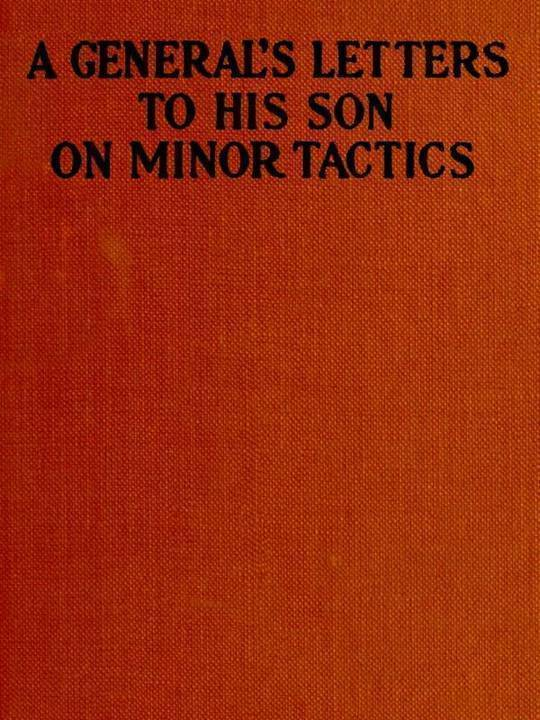Anonymous
A General's Letters to His Son on Minor Tactics
Free
Description
Contents
Reviews
Language
English
ISBN
Unknown
CONTENTS
A GENERAL’S LETTERS TO HIS SON ON MINOR TACTICS
PREFACE
A GENERAL’S LETTERS TO HIS SON ON MINOR TACTICS
LETTER I
LETTER II
Problem 1
Problem.
Action considered Correct.
Orders.
Problem.
Action considered Correct.
Orders.
Problem 1
Problem.
Action considered Correct.
Orders.
Problem.
Action considered Correct.
Orders.
LETTER III
Problem 2
Solutions.
Solutions.
Problem 2
Solutions.
Solutions.
LETTER IV
Problem 3
Question 1.
Action considered Correct.
Question 2.
Action which is considered Correct.
Question 3.
Action considered Correct.
Question 1.
Action considered Correct.
Question 2.
Action which is considered Correct.
Question 3.
Action considered Correct.
Problem 3
Question 1.
Action considered Correct.
Question 2.
Action which is considered Correct.
Question 3.
Action considered Correct.
Question 1.
Action considered Correct.
Question 2.
Action which is considered Correct.
Question 3.
Action considered Correct.
LETTER V
Problem 4
Problem.
Solution considered Correct.
Orders.
Problem.
Solution considered Correct.
Orders.
Problem 4
Problem.
Solution considered Correct.
Orders.
Problem.
Solution considered Correct.
Orders.
LETTER VI
Problem 5a
Action considered Correct.
Action considered Correct.
Problem 5b
Action considered Correct.
Action considered Correct.
Problem 5c
Action considered Correct.
Action considered Correct.
Problem 5a
Action considered Correct.
Action considered Correct.
Problem 5b
Action considered Correct.
Action considered Correct.
Problem 5c
Action considered Correct.
Action considered Correct.
LETTER VII
Problem 6
Solution considered Correct.
Solution considered Correct.
Problem 6
Solution considered Correct.
Solution considered Correct.
LETTER VIII
Problem 7
Problem.
Solution considered Correct.
Problem.
Solution considered Correct.
Problem 8
Solution considered Correct.
Solution considered Correct.
Problem 7
Problem.
Solution considered Correct.
Problem.
Solution considered Correct.
Problem 8
Solution considered Correct.
Solution considered Correct.
LETTER IX
Problem 9
Solution.
Solution.
Problem 9
Solution.
Solution.
LETTER X
Problem 10
Problem.
Solution.
Problem.
Solution.
Problem 10
Problem.
Solution.
Problem.
Solution.
LETTER XI